## Multivariate maps

Multivariate plots summarizing composition of pinch-point buffers.

### Slope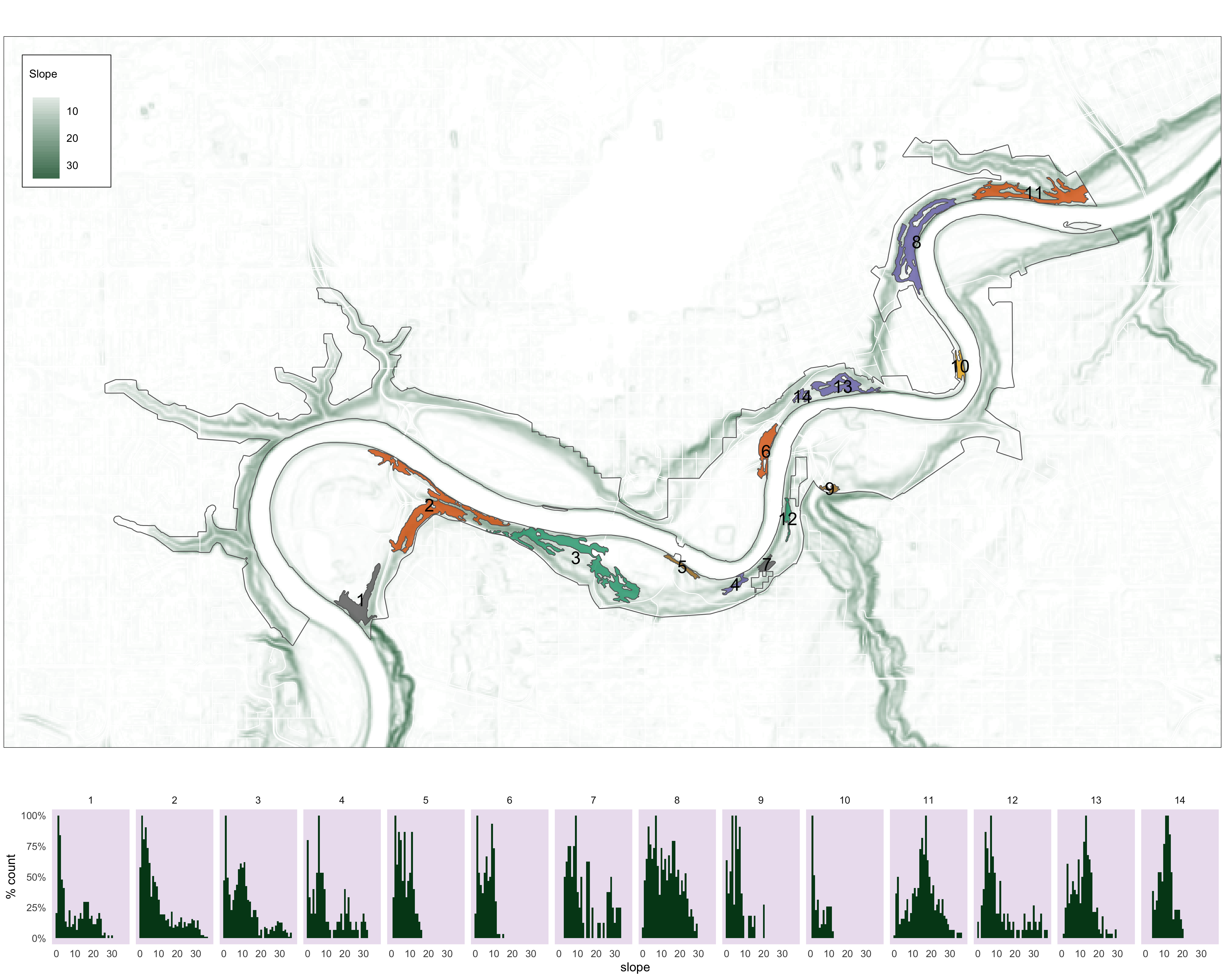Figure 4.25: Histograms of slope values for each pinch point.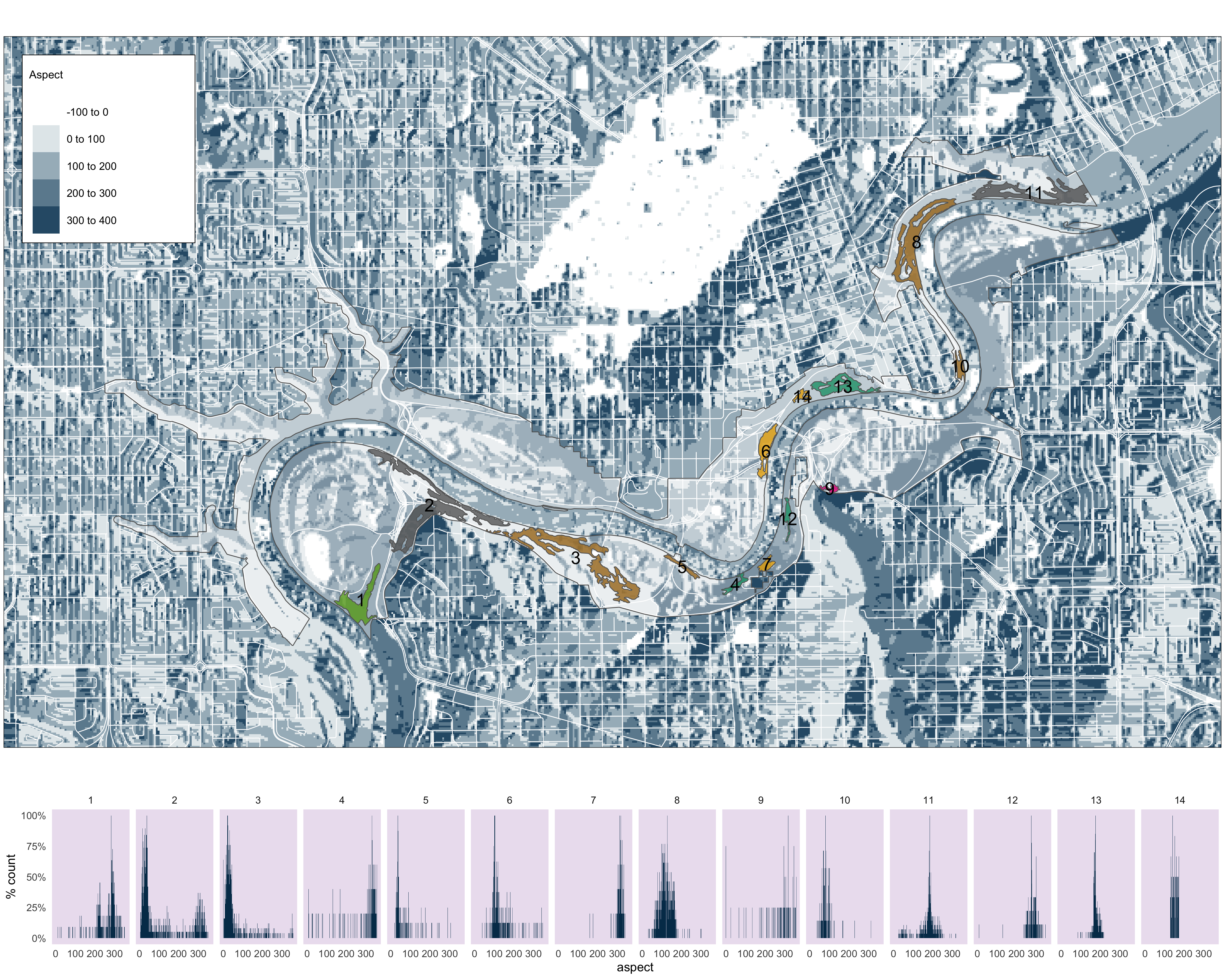Figure 4.26: Histograms of aspect values for each pinch point.

### Vegetation cover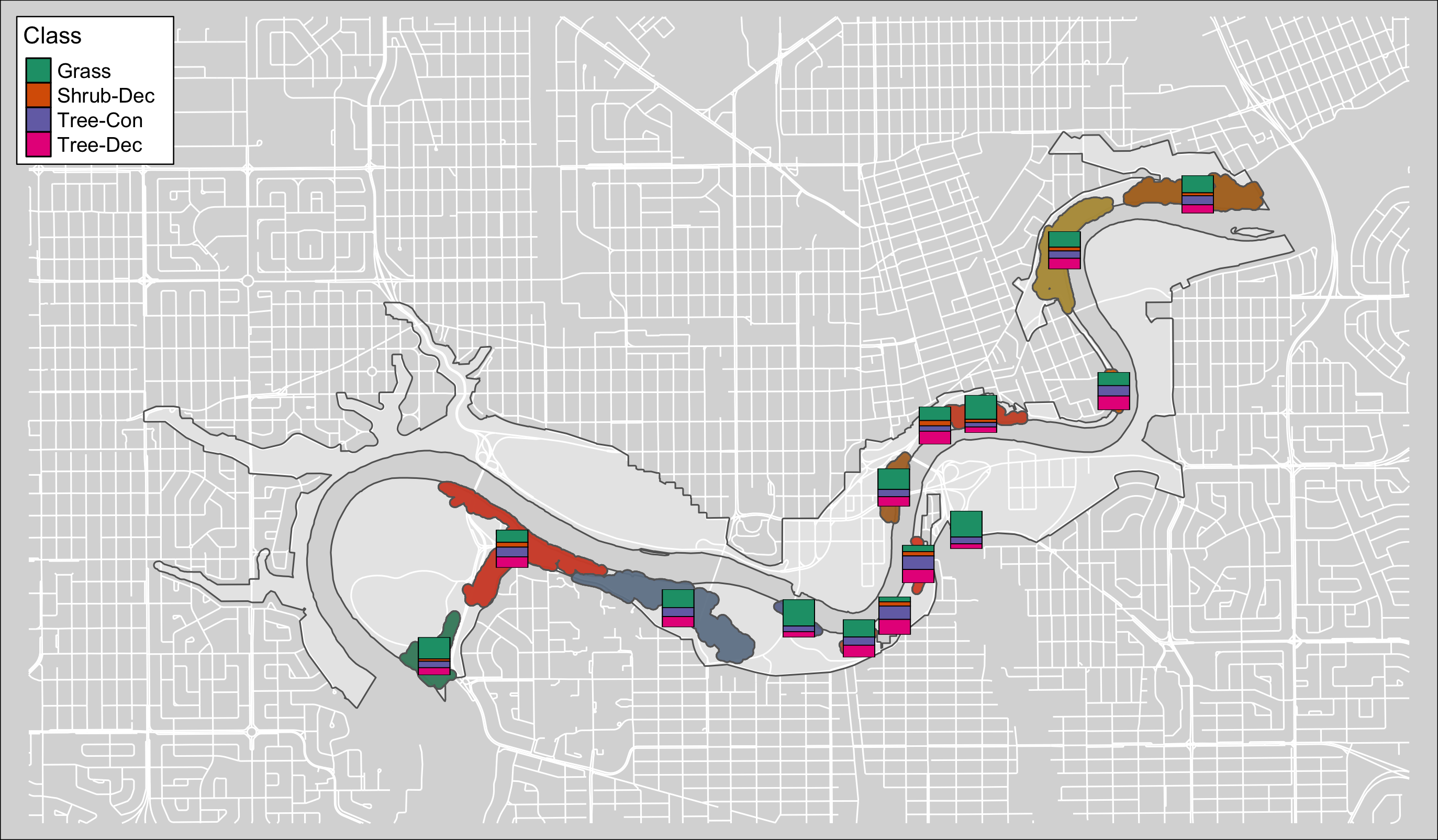Figure 4.27: Canopy poly class map #1.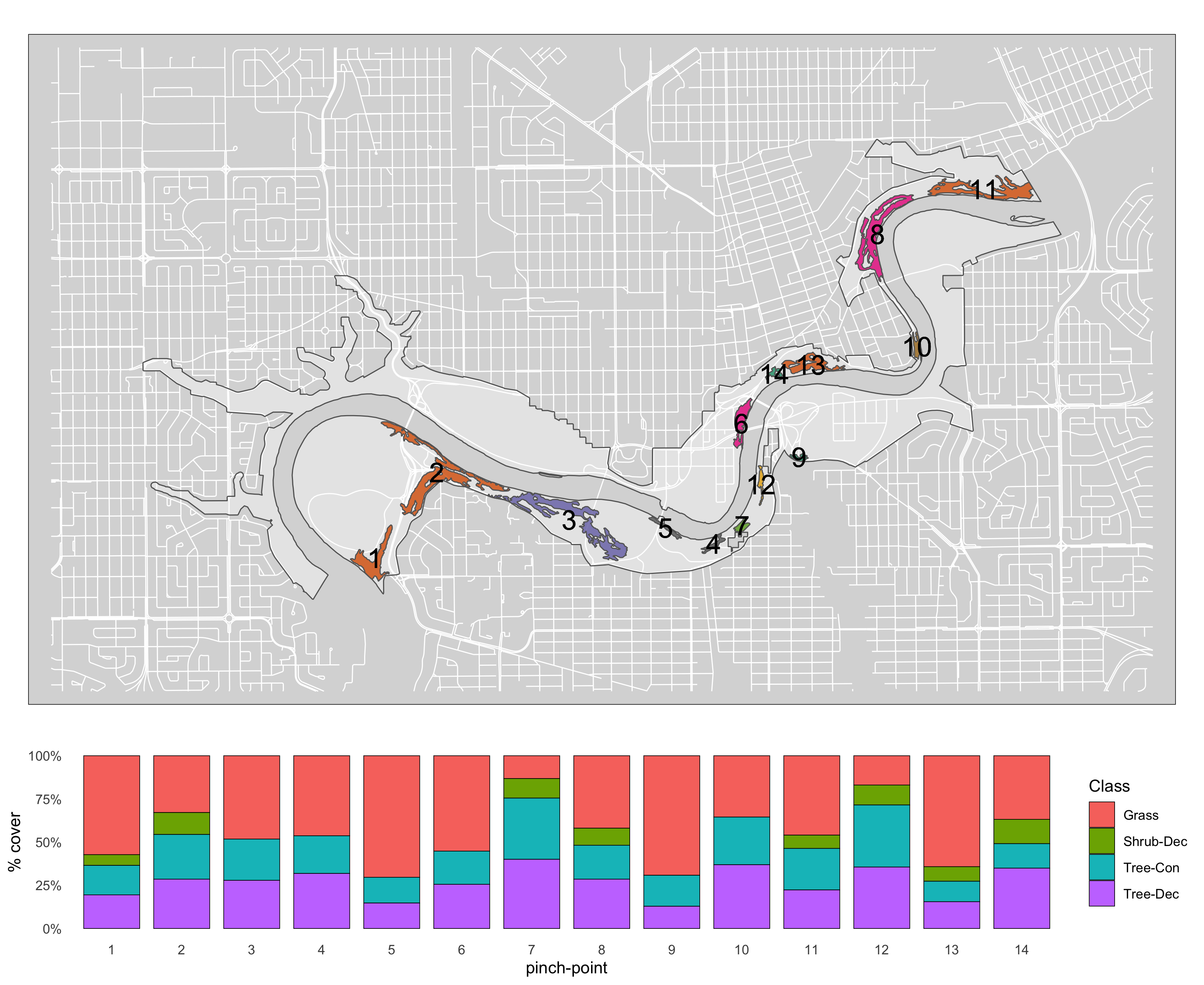Figure 4.28: Canopy poly class map #2.

### Landcover

Data was from the UPLVI layer.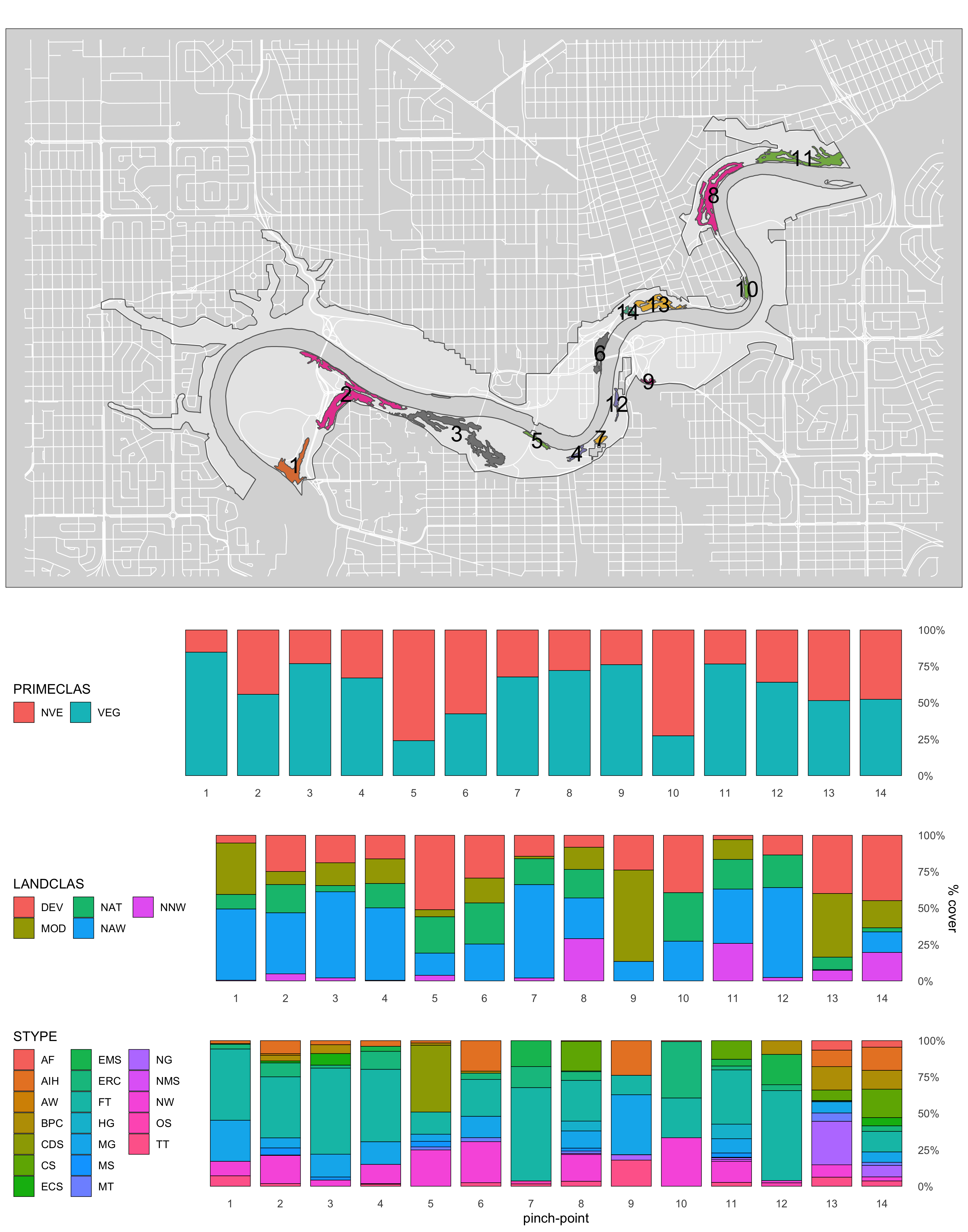Figure 4.29: Canopy poly class map #2.

### 4.0.2 Linear features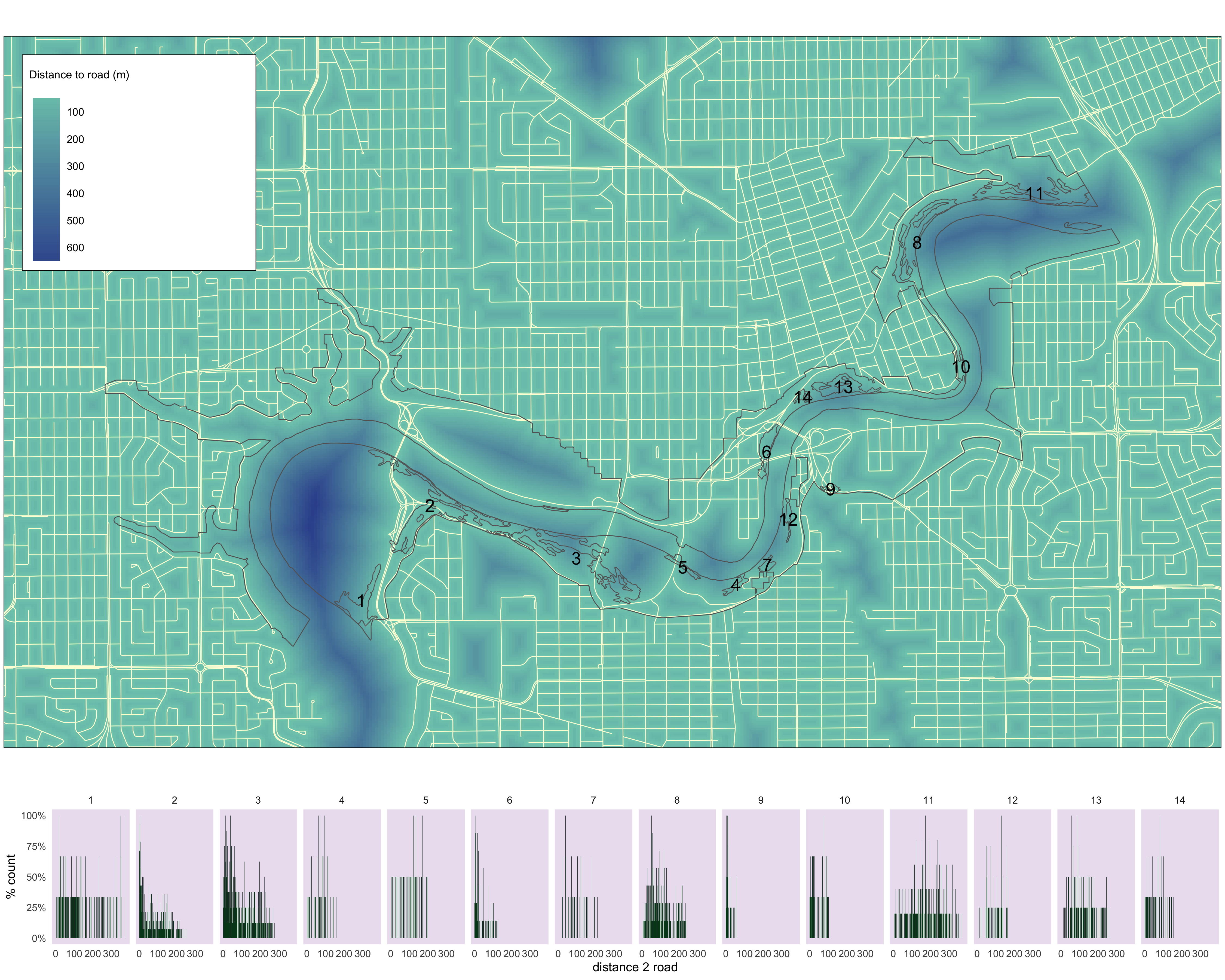Figure 4.30: Histograms of distance to road values for each pinch point.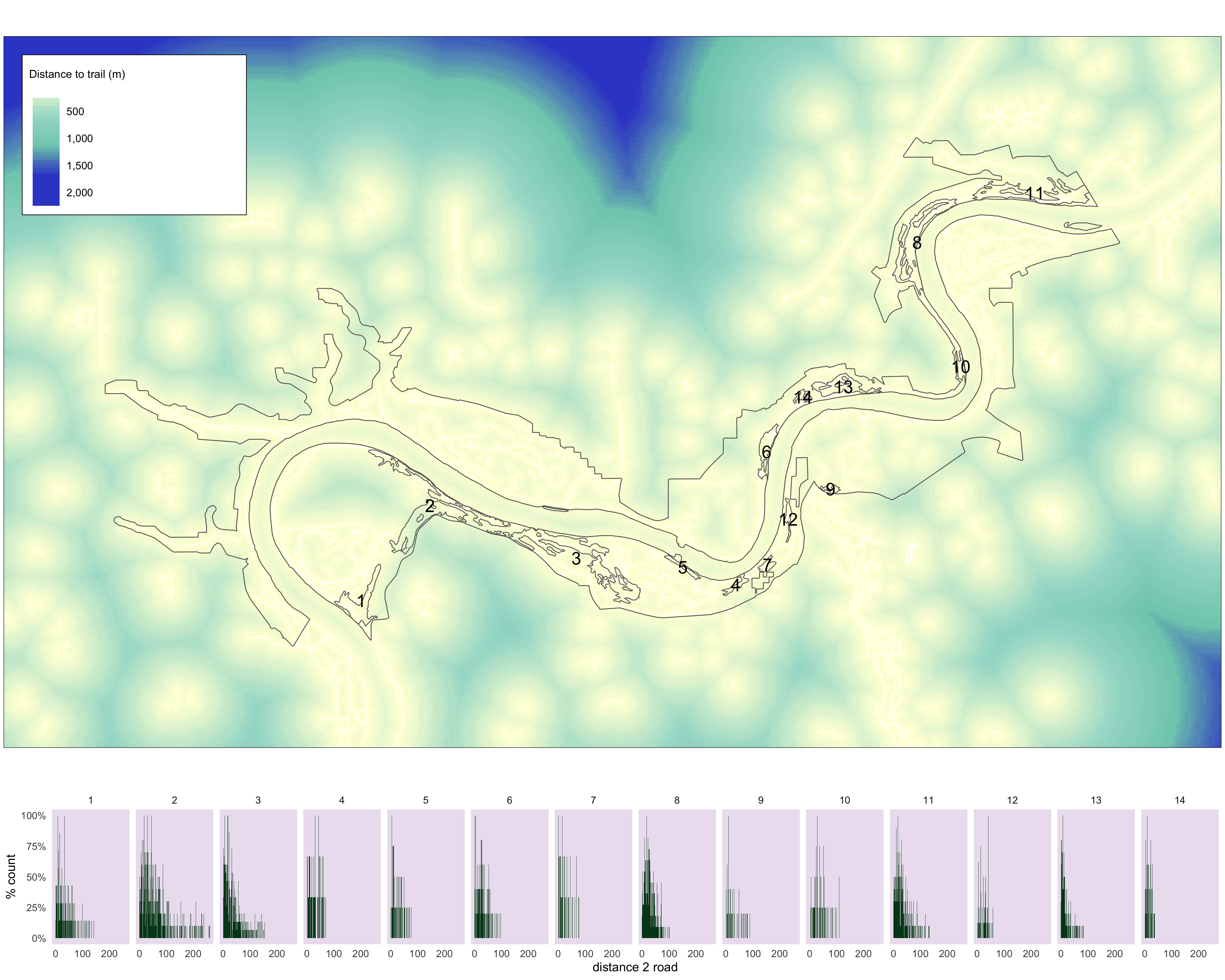Figure 4.31: Histograms of distance to trail values for each pinch point.